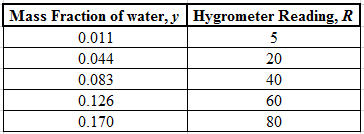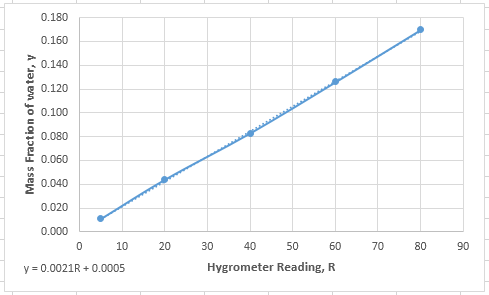# 2.40. WP A hygrometer, which measures the amount of moisture in a gas stream, is to be calibrated using the apparatusshown here:AirDRYERROTAMETERSHYGROMETERWaterEVAPORATORSteamSteam and dry air are fed at known flow rates and mixed to form a gas stream with a known water content, and the hygrometerreading is recorded; the flow rate of either the water or the air is changed to produce a stream with a different water content andthe new reading is recorded, and so on. The following data are taken:Mass Fraction of Water, y HygrometerReading, R0.0110.044200.083400.126600.17080a. Draw a calibration curve and determine an equation for y(R).b. Suppose a sample of a stack gas is inserted in the sample chamber of the hygrometer and a reading of R = 43 is obtained. If themass flow rate of the stack gas is 1200 kg/h, what is the mass flow rate of water vapor in the gas?AnswerBIOENGINEERING110111111111111011 2.42. WP The temperature in a process unit is controlled by passing cooling water at a measured rate through a jacket thatencloses the unit.Cooling waterPROCESSUNITROTAMETERp(liters/s)T(°C)JacketThe exact relationship between the unit temperature T(°C) and the water flow rate o (L/s) is extremely complex, and it is desiredto derive a simple empirical formula to approximate this relationship over a limited range of flow rates and temperatures. Data aretaken for T versus ø. Plots of T versus ø on rectangular and semilog coordinates are distinctly curved (ruling out T = aø + b andT = aebó as possible empirical functions), but a log plot appears as follows:300T(°C) 20010010100p(L/s)A line drawn through the data goes through the points (ø1 = 25 L/s, T = 210°C) and (ø2 = 40 L/s, T, = 120°C).a. What is the empirical relationship between o and T?Answerb. Using your derived equation, estimate the cooling water flow rates needed to maintain the process unit temperature at 85°C,175°C, and 290°C.c. In which of the three estimates in Part (b) would you have the most confidence and in which would you have the leastconfidence? Explain your reasoning.

Question
4 views

The problems that are listed below need to be solved and you may access those problems via viewing them through the attached images in this request. To emphasize more, the problems are 2.40; 2.42.

check_circle

Step 1

Note: Since we are entitled to answer only the first question, we’ll answer 2.35. Please resubmit the other questions separately which you’d like answered.

Step 2

(a)

The data that were taken for the hygrometer reading, R and mass fraction of water, y is given as:Step 3

Plot this data on a graph and fit the curve in a straight line. Use the trendline tool in excel and fit the data in a linear equation as shown below:...

### Want to see the full answer?

See Solution

#### Want to see this answer and more?

Solutions are written by subject experts who are available 24/7. Questions are typically answered within 1 hour.*

See Solution
*Response times may vary by subject and question.
Tagged in